Published: 26 September 2017

# Noise and vibration exposure of hybrid PEM power supply users during its operation

Maciej Kłaczyński1
Grzegorz Grzeczka2
1AGH University of Science and Technology, Department of Mechanics and Vibroacoustics, Krakow, Poland
2Polish Naval Academy, Institute of Electrical Engineering and Automation, Gdynia, Poland
Corresponding Author:
Maciej Kłaczyński
Views 54

#### Abstract

Proton exchange membrane (PEM) fuel cells characterized the highest available current density of electricity, but their construction uses elements, which generate noise, vibration and requires the dissipation of heat. Emitting these physical fields into the environment from this type of power sources is a disadvantage due to the low detection requirements of military installations as well as operator safety. The paper presents the study of noise and vibration exposure users of 20 Watt PEM fuel cell users.

## 1. Introduction

Each of the existing and developing military areas is dependent on electricity sources. Military requirements such as dimensions, operating conditions, power range and generated physical fields are very specific. It follows from theoretical analysis of technology used in primary and secondary batteries, that they would not achieve the desired value in military applications, when used as an independent power source in many applications. Alternative power sources are the available solution in order to comply with the said requirements. Such alternative sources can replace batteries completely or in a hybrid system, where they may improve efficiency of the entire system. The most commonly considered portable source of electricity are low temperature polymer fuel cells, well knows as PEM fuel cell. They are characterized by the highest available current density of electricity, but their construction uses elements, which generate noise and vibration, as a principle of operation requires the dissipation of heat. Emitting the said physical fields into the environment from power sources is a disadvantage due to the low detection requirements of military installations as well as operator’s safety. The paper presents the study of noise and vibration exposure users of hybrid power supply with a 20 Watt PEM fuel cell.

## 2. Hybrid power supply source

Hybrid power supply source is a device supporting the rescue and evacuation action (Fig. 1) enabling a rescue team to react in a crisis without the need to supply electricity or fuel for its production. The basis of the project was the original electric and mechanic idea of electric energy storage which born in Polish Naval Academy, Institute of Electrical Engineering and Automation. This solution consisting of individual power units of a 20 Watt rescuer that can connect to the master system (Fig. 2) and when can be power supply of field hospitals, crisis centres, lighting, communications devices, tools etc.

## 3. Noise and vibration exposure regulation

Member States of the European Union are obliged to implement into the internal legal system provisions of the following EU Directives: 2003/10/EC (dated 6 February 2003)  and 2002/44/EC (dated 25 June 2002)  on the minimum health and safety requirements regarding the exposure of workers to the risks arising from physical agents such as noise and vibration. The main requirement of these Directives is establishment of a clear and coherent prevention strategy, which would safeguard workers’ health and safety, when exposed to noise and vibration.

Fig. 120W PEM hybrid power supply module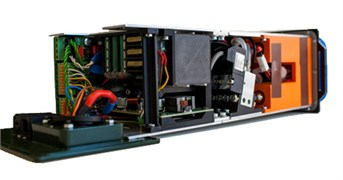Fig. 2Master system of 20W PEM hybrid power supply module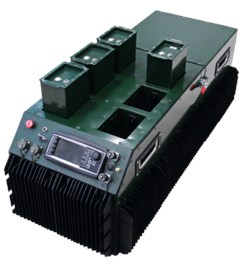### 3.1. Provisions of the directive 2003/10/EC

In order to prevent hearing damage to workers, the Directive establishes limits on exposure to noise, i.e.:

– exposure level: ${L}_{Ex,8h}=$ 87 dB(A),

– peak sound pressure ${P}_{peak}=$ 200 Pa (what suits peak sound pressure level ${L}_{Cpeak}=$ 140 dB(C) re. 20 μPa).

Where:

– peak sound pressure (${P}_{peak}$): maximum value of the ‘$C$’ – frequency weighted instantaneous noise pressure,

– daily noise exposure level (${L}_{EX,8h}$) (dB(A) re. 20 μPa): timeweighted average of the noise exposure levels for a nominal eight-hour working day as defined by international standard ISO 1999: 1990, point 3.6. It covers all noises present at work, including impulsive noise.

According to this Directive, no worker is allowed to exposure to higher values, and the noise reaching to his ear should be kept below these values. The Directive also establishes upper and lower action values, at whose employer is required to take preventive action.

Exposure action values in respect of the daily noise exposure levels and peak sound pressure are fixed at:

${L}_{Ex,8h}=$ 85 dB and ${P}_{peak}=$ 140 Pa (${L}_{Cpeak}=$ 137 dB re. 20 μPa) – upper values,

${L}_{Ex,8h}=$80 dB and ${P}_{peak}=$ 112 Pa (${L}_{Cpeak}=$ 135 dB re. 20 μPa) – lower values.

The precautionary measures set up by Directive include, in particular, designation and the occupational risk assessment made by the employer using different methods for assessing the level of exposure to noise and taking into account the circumstances of exposure. Therefore, in order to correctly assess worker exposure to noise using an objective measurement method, the Directive generally refers to ISO 1999: 1990 .

Important that, when applying the exposure limit values, the determination of the worker’s effective exposure shall take account of the attenuation provided by the individual hearing protectors worn by the worker. The exposure action values shall not take account of the effect of any such protectors. However, in not only authors’ opinion, it would be advisable also to refer to ISO 9612: 2009 .

### 3.2. Provisions of the directive 2002/44/EC

In order to prevent harmful effects of vibration on the health and safety of workers, the Directive establishes exposure limit values and action values at which the employer is required to take specific preventive measures.

For hand-arm vibration:

– the daily exposure limit value standardised to an eight-hour reference period shall be A(8)h = 5 m/s2,

– the daily exposure action value standardised to an eighthour reference period shall be A(8)h = 2,5 m/s2.

The assessment of the level of exposure to hand-arm vibration is based on the calculation of the daily exposure value normalised to an eight-hour reference period A(8)h, expressed as the square root of the sum of the squares (rms) (total value) of the frequency-weighted acceleration values, determined on the orthogonal axes ${a}_{hwx}$, ${a}_{hwy}$, ${a}_{hwz}$ as defined in Chapters 4 and 5 and Annex A to ISO standard 5349-1(2001)  (see Eq. (1)):

1
${a}_{hv}=\sqrt{{a}_{hwx}^{2}+{a}_{hwy}^{2}+{a}_{hwz}^{2}}.$

For whole-body vibration:

– the daily exposure limit value standardised to an eight-hour reference period shall be A(8)w = 1,15 m/s2 or, at the choice of the Member State concerned, a vibration dose value (VDV) of 21 m/s1,75;

– the daily exposure action value standardised to an eight-hour reference period shall be 0,5 m/s2 or, at the choice of the Member State concerned, a vibration dose value (VDV) of 9,1 m/s1,75.

The assessment of the level of exposure to vibration is based on the calculation of daily exposure A(8) expressed as equivalent continuous acceleration over an eight-hour period, calculated as the highest (rms) value, or the highest vibration dose value (VDV) of the frequency-weighted accelerations, determined on three orthogonal axes (1.4${a}_{wx}$, 1.4${a}_{wy}$, ${a}_{wz}$ for a seated or standing worker) in accordance with Chapters 5, 6 and 7, Annex A and Annex B to ISO standard 2631-1(1997)  (see Eq. (2)):

2
${a}_{wv}=\sqrt{{1.4a}_{wx}^{2}+{1.4a}_{wy}^{2}+{a}_{wz}^{2}}.$

The VDV formulae uses the RMS Acceleration raised to the fourth power and is known as the Root-mean-quad method. This technique ensures the VDV is more sensitive to the peaks in the acceleration levels according Eq. (3):

3
$VDV={\left(\underset{0}{\overset{T}{\int }}{a}^{4}\left(t\right)dt\right)}^{1/4},$

where: VDV is the vibration dose value in m/s1.75, $a\left(t\right)$ is the frequency weighted acceleration in m/s2, $T$ is the total measurement period in seconds.

However, in not only authors’ opinion, for whole-body vibration measurements and the level of exposure to vibration estimation, it would be advisable also to refer to PN-EN 14253 (2003) .

According to valid regulations, the correction filters used in the measurements instruments are to meet the requirements of ISO 8041 . These are filters shown in Table 1.

## 4. Noise and vibration tests of 20 W hybrid PEM power supply

Measurements of the operation of the device were performed in a specialized research facility - in an anechoic chamber, at the Department of Mechanics and Vibroacoustic, AGH University of Science and Technology, Krakow, Poland. Measurement conditions met the requirements for acoustic power measurements according to . Four-channel sound and vibration level meter SVAN 958 equipped with the 1/2 type 40AZ free field microphone from G.R.A.S with the SVANTEK SV 12L preamplifier and PCB Piezotronics 3-Axis Type 356B18 vibration sensor, were used. This instrument allows measurements in class 1 sound pressure level, i.e. – ${L}_{max}$, ${L}_{min}$, ${L}_{eq}$, SEL, ${L}_{peak}$ with selected correction filter A, C, Z and FAST time constant, as well as measurement of 1/1 or 1/3 octave bands and measurement of vibration acceleration parameters - RMS, PP, Peak, Max, with selected correction filter HP, ${W}_{k}$, ${W}_{d}$, ${W}_{m}$, ${W}_{h}$ independently in three axes (${a}_{x}$, ${a}_{y}$, ${a}_{z}$). The measurement coherence ensured the acoustic calibration of the measured track with the SVANTEK reference acoustic source type SV30A with SPL level 113.9 dB and the Bruel & Kjaer reference vibration source type 4294 with an acceleration level of 10 m/s2.

Table 1Type of correction filters for vibration exposure assessments according ISO 8041

 Type of vibration impact Type of characteristics Frequency band [Hz] For whole-body vibration (basic characteristics) Vertical direction ${W}_{k}$ 0.5-80 Horizontal direction ${W}_{d}$ 0.5-80 Omnidirectional (in buildings) ${W}_{m}$ 1-80 For whole-body vibration (extra characteristics) For horizontal direction on a seat back rest ${W}_{c}$ 0.5-80 Vertical recumbent (head) ${W}_{j}$ 0.5-80 For hand-arm vibration ${W}_{h}$ 8-1000

Fig. 3Unweighted average (5 minutes of work) spectrum of the sound power level generated by 20 W PEM hybrid power supply module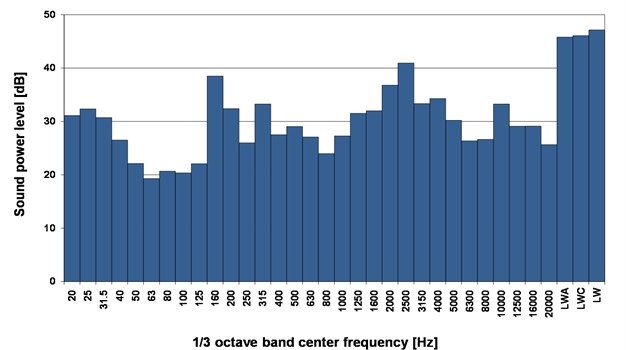As a result of the vibroacoustic study of a 20 W PEM hybrid power supply module, it was found that its acoustic power was ${L}_{WA}=$ 45 dB(A). This means that the sound pressure level at close range (up to 1 m) will not be greater than ${L}_{Aeq}=$ 51 dB(A). During tests, largest measured peak level was ${L}_{Cpeak}=$ 87.1dB(C). The average (5 minutes of work) spectrum of sound power level is presented at Fig. 3. Sound pressure level (${L}_{eq}$) and A-weighted sound pressure level (${L}_{Aeq}$) courses for half minute of work are shown at Fig. 4. Acceleration parameters of vibration for HP filter (frequency band 0.5-3000 Hz) respectively are:

RMS: ${a}_{x}=$0.25 m/s2, ${a}_{y}=$0.32 m/s2, ${a}_{z}=$0.16 m/s2;

MAX: ${a}_{x}=$0.32 m/s2, ${a}_{y}=$0.39 m/s2, ${a}_{z}=$0.25 m/s2;

PEAK: ${a}_{x}=$4.8 m/s2, ${a}_{y}=$4.1 m/s2, ${a}_{z}=$6.7 m/s2;

P-P: ${a}_{x}=$8.93 m/s2, ${a}_{y}=$7.3 m/s2, ${a}_{z}=$12.26 m/s2.

Unweighted (HP filter) RMS acceleration courses (${a}_{x}$, ${a}_{y}$, ${a}_{z}$) generated by 20 W PEM hybrid power supply module are presented at Fig. 5. Usage and assembly of 20W PEM hybrid power supply module indicate that the assessment of the level of exposure for vibration concerns whole body. Made measurements and calculations indicate that, the exposer level is A(8)w = 0.12 m/s2 and vibration dose value is $VDV=$ 3.3 m/s1.75.

Fig. 4Sound pressure level – Leq, A-weighted sound pressure level – LAeq courses generated by 20W PEM hybrid power supply module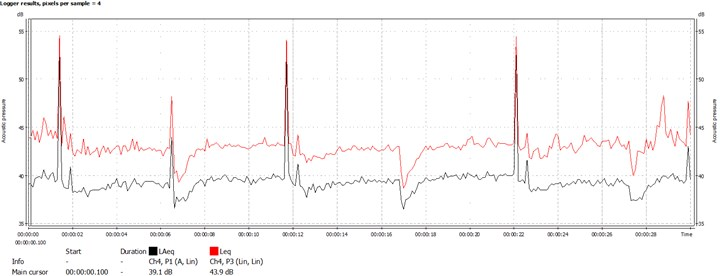Fig. 5Unweighted (HP filter) RMS acceleration courses (ax, ay, az) generated by 20 W PEM hybrid power supply module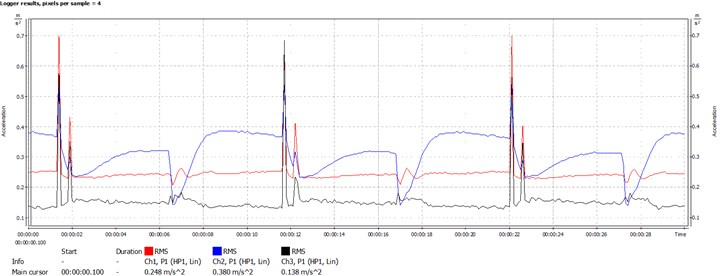## 5. Conclusions

As a result of the tests it was stated that the permissible levels of noise and vibration exposure were not exceeded. Thus, the device is safe for the user to be at risk of noise and vibration.

#### Cited by

Doping of scandia-stabilized zirconia electrolytes for intermediate-temperature solid oxide fuel cell: A review

06 September 2017
Accepted
07 September 2017
Published
26 September 2017
SUBJECTS
Acoustics, noise control and engineering applications
Keywords
noise and vibration exposure
personal power source
PEM fuel cell
Acknowledgements

The research project referred to in this paper was implemented within the framework of Project No. DOBR /0061/R/ID2/2012/03 (Polish Naval Academy) and statutory research (AGH University of Science and Technology).